• 给定一个n个点m条边的有向图，点的编号是1到n，图中可能存在重边和自环。 请输出任意一个该有向图拓扑序列，如果拓扑序列不存在，则输出-1。 若一个由图中所有点构成的序列A满足：对于图中的每条边(x, y)，x在A...
思路：
用数组模拟链表，首先如果能用拓扑排序则其一定是有向无环图。
所以我们应该先找到其入度为0的点。然后将其入队，并将与此点相连的边全部删去。最后当队尾指针是n-1时说明一共入队n个元素说明此图是有向无环图。存在拓扑排序。
注意：h[i]中存储的是元素在e[]中的下标。
问题描述
给定一个n个点m条边的有向图，点的编号是1到n，图中可能存在重边和自环。
请输出任意一个该有向图的拓扑序列，如果拓扑序列不存在，则输出-1。
若一个由图中所有点构成的序列A满足：对于图中的每条边(x, y)，x在A中都出现在y之前，则称A是该图的一个拓扑序列。
输入格式
第一行包含两个整数n和m
接下来m行，每行包含两个整数x和y，表示存在一条从点x到点y的有向边(x, y)。
输出格式
共一行，如果存在拓扑序列，则输出任意一个合法的拓扑序列即可。
否则输出-1。
数据范围
1≤n,m≤105
输入样例：
3 3
1 2
2 3
1 3
输出样例：
1 2 3
代码
#include<iostream>
#include<cstring>
using namespace std;
const int N = 100010;
int e[N], ne[N], h[N], idx = 0;
int q[N], d[N];
int n = 0, m = 0;
e[idx] = b, ne[idx] = h[a], h[a] = idx++;
}

bool toposort()
{

int hh = 0, tt = -1;
for (int i = 1; i <= n; ++ i){
if (!d[i]){
q[++ tt] = i;
}
}
while(hh <= tt){
int t = q[hh++];
for(int i = h[t]; i != -1; i = ne[i]){
d[e[i]]--;
if(!d[e[i]])
q[++tt] = e[i];
}
}
return tt == n-1;
}

int main(){
cin >> n >> m;
int a, b;
memset(h, -1, sizeof h);
for(int i = 0; i < m; ++i){
cin >> a >> b;
d[b]++;
}
if(toposort()){
for(int i = 0; i <= n - 1; ++i){
cout << q[i] << " ";
}
}else{
cout << "-1" << endl;
}
return 0;
}

原题链接


展开全文链表 算法
• 不含环路的有向图必包含入度为零的顶点—因此一定存在拓扑排序 拓扑排序的应用 图是否存在环 拓扑排序具体算法 拓扑排序是将有向无环图G的所有顶点排成一个线性序列， 使得对图G中的任意两个顶点u、v，如果存在...

转载自C++ 拓扑排序算法

有向无环图

如果一个有向图的任意顶点都无法通过一些有向边回到自身，那么称这个有向图为有向无环图。

不含环路的有向图必包含入度为零的顶点—因此一定存在拓扑排序
拓扑排序的应用

图是否存在环

拓扑排序具体算法

拓扑排序是将有向无环图G的所有顶点排成一个线性序列，
使得对图G中的任意两个顶点u、v，如果存在边u->v，那么在序列中u一定在v前面，
这个序列又被称为拓扑序列。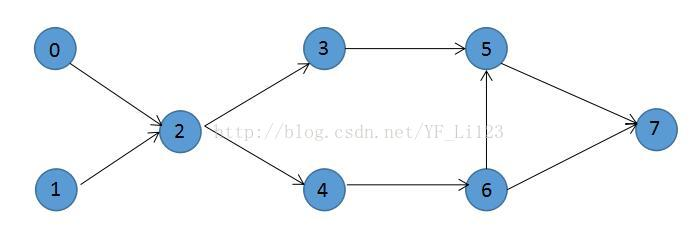结点0和1没有前驱节点，可以任意访问，
但是结点2必须在结点0和1访问完之后才能访问，同理结点3和4必须在结点2访问完以后才能访问，
但是结点3和4 之间没有依赖关系，结点5必须在结点3和结点6访问之后才能访问，等等…
因此，对于这个图的一个拓扑序列可以是：0,1,2,3,4,6,5,7 也可以是：0,1,2,4,6,3,5,7

拓扑排序的步骤
步骤如下:

定义一个队列Q,把入读为0的节点加入队列.
取出队首元素进行访问,然后删除所有从它出发的边.并加能到达的顶点的入度-1.若这个点的入度为0,那么就将其加入队列.
重复(2)的操作,直到队列为空. 若队列为空,且访问过N个顶点了.就说明拓扑排序成功.图G为有向无环图.否则.拓扑排序失败.图G有环.

代码实现
/*
拓扑排序:
1. G邻接表
2. n个顶点
3. 入度
*/
bool TopSort(vector<vector<int>> &G, int n, vector<int> &inDegree) {

vector<int> res;

int cnt = 0; // 记录加入图片排序的顶点数

queue<int> q;
for (int i = 0; i < n; i++) {
if (inDegree[i] == 0) {
// 入度为0,入队
q.push(i);
}
}

while (!q.empty()) {
// 取队首
int from = q.front(); q.pop();
// 访问from
res.push_back(from);

// 遍历邻接表:它能到的顶点
for (int i = 0; i < G[from].size(); i++) {
int to = G[from][i];
if (--inDegree[to]==0) {
q.push(to);
}
}
// 把form能到的边全部清空
//G[from].clear();
cnt++;
}

for (auto x : res) {
cout << x << ends;
}
cout << endl;

if (cnt == n) {
return true;
}
return false;
}

int main() {

int n, m;
cout << "请输入顶点数和边数:";
cin >> n >> m;
vector<vector<int>> G(n);
for (int i = 0; i < m; i++) {
int x, y;
cout << "请输入第" << i + 1 << "条边的顶点:";
cin >> x >> y;
G[x].push_back(y);
}
cout << "拓扑排序为:";
vector<int> inDegree(n);
for (auto x : G) {
for (auto y : x)
inDegree[y]++;
}
bool res = TopSort(G, n, inDegree);

system("pause");
return 0;
}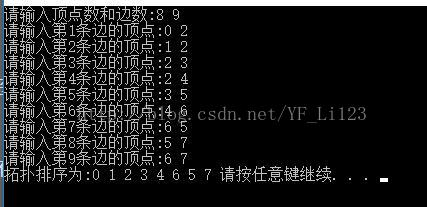应用
家族辈分


展开全文• ## 有向图的拓扑排序

万次阅读 多人点赞 2016-03-15 23:01:41
有向图拓扑排序的基本思想是：首先在有向图中选取一个没有前驱的顶点，将其输出，从有向图中删除该顶点，并且删除以该顶点为尾的所有有向图的边。重复以上的步骤，直到图中的所有顶点均输出或是图中的顶点均没有...
                                                                               有向图的拓扑排序

本文取自《数据结构与算法》(C语言版)(第三版)，出版社是清华大学出版社。
本博文作为学习资料整理。源代码是VC++ 6.0上可执行程序，我挪到了VS2010中执行。

在VS2010中新建C++ Win32 控制台应用程序项目，创建结果截图：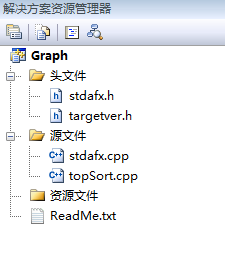有向图的拓扑排序的基本思想是：首先在有向图中选取一个没有前驱的顶点，将其输出，从有向图中删除该顶点，并且删除以该顶点为尾的所有有向图的边。重复以上的步骤，直到图中的所有顶点均输出或是图中的顶点均没有前驱为止。对于后者，说明有向图中存在环，不能进行拓扑排序。
以下以AOV(Activity On Vertex Network)网为例，演示求解拓扑排序的步骤：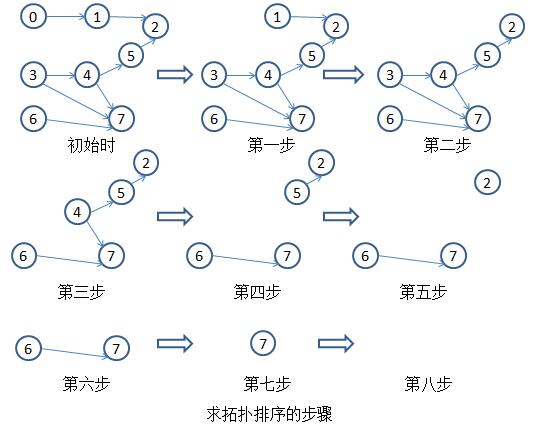C++ Win32 控制台应用程序，代码如下：topSort.cpp

  #include "stdafx.h"
#include<stdio.h>
#include<string.h>
#define MAXNODE 20
typedef struct
{
char vertex;
}VerNode;

typedef struct
{
}Arc;

typedef struct
{
VerNode vexs[MAXNODE];
Arc arcs[MAXNODE][MAXNODE];
int m_numOfVexs;
}Graph;

int mFind(char aim, VerNode* arry)
{
int pos=-1;
int i=0;
for(i=0; i<MAXNODE; i++)
{
if(aim==arry[i].vertex)
{
pos=i;
return pos;
}
}
return pos;
}

void showGraph(Graph aGraph, int num)
{
int i=0;
int j=0;
printf("\n NODES:\n");
for(i=0; i<num; i++)
{
printf(" %c", aGraph.vexs[i].vertex);
}
printf("\n ARCS:\n");
for(i=0; i<num; i++)
{
for(j=0; j<num; j++)
{
}
printf(" \n");
}
}

//添加顶点
{
aGraph->m_numOfVexs++;
aGraph->vexs[aGraph->m_numOfVexs].vertex=vex;
}

//添加边
void addArcs(Graph* aGraph, char aStart, char aEnd)
{
int p_x=mFind(aStart,aGraph->vexs);
int p_y=mFind(aEnd,aGraph->vexs);
}

//图的初始化
void initGraph(Graph* aGraph)
{
int i=0;
int j=0;
aGraph->m_numOfVexs=-1;
for(i=0; i<MAXNODE; i++)
{
for(j=0; j<MAXNODE; j++)
}
}

int getInDegree(int i, Graph* aGraph)
{
int InDegree=0;
int j=0;
for(j=0; j<aGraph->m_numOfVexs; j++)
return InDegree;
}

int topSort(Graph* aGraph)
{
int i;
int isOK=1;
int vexsIsOut[MAXNODE];
for(i=0; i<aGraph->m_numOfVexs; i++)
{
vexsIsOut[i]=0;
}
while(isOK==1)
{
isOK=0;
for(i=0; i<aGraph->m_numOfVexs; i++)
{
if(vexsIsOut[i]==0&&getInDegree(i,aGraph)==0)
{
int j;
printf("%c ",aGraph->vexs[i].vertex);
vexsIsOut[i]=1;
for(j=0; j<aGraph->m_numOfVexs; j++)
{
}
isOK=1;
}
}
}
for(i=0; i<aGraph->m_numOfVexs; i++)
{
if(vexsIsOut[i]==0)
return 0;
}
return 1;
}

int main(void)
{
char node='a';
char input1='a';
char input2='a';
//将图初始化
Graph g_graph;
initGraph(&g_graph);

//根据用户的输入添加顶点
printf("Please input the vexs( end with #):\n");
while(1)
{
if(node=='#')
break;
if(scanf("%c,",&node)==1)
{
if(node=='\n')
continue;
}
}

//根据用户的输入添加边
printf("Please input arcs, just like this \"startNod,endNode\" \n");
while(1)
{
if(input1=='#')
break;
if(scanf("%c,%c",&input1, &input2)==2)
{
if(input1=='\n'||input2=='\n')
continue;
}
}

//输出图
showGraph(g_graph, g_graph.m_numOfVexs);

printf("The topsort is: \n");
if(topSort(&g_graph)==0)
printf("There is a circle in the graph!! \n");
return 0;
}


Ctrl+F5执行topSort.cpp代码，结果如下图：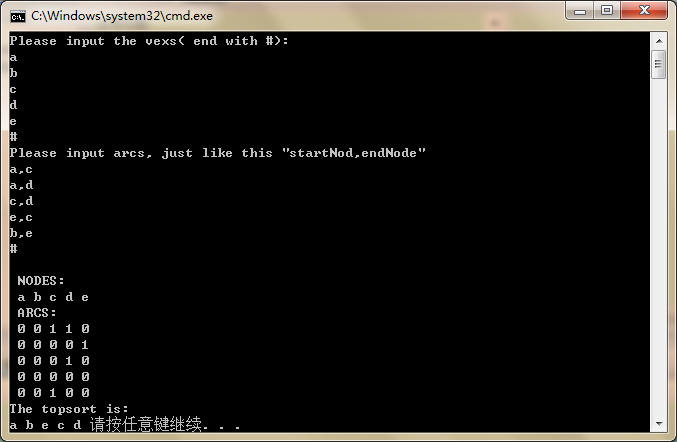展开全文图算法 C
• 请输出任意一个该有向图拓扑序列，如果拓扑序列不存在，则输出−1。 若一个由图中所有点构成的序列AA满足：对于图中的每条边(x,y)，x在A中都出现在y之前，则称A是该图的一个拓扑序列。 输入格式 第一行包含两个...
题目

给定一个 n 个点 m 条边的有向图，点的编号是 1 到 n，图中可能存在重边和自环。

请输出任意一个该有向图的拓扑序列，如果拓扑序列不存在，则输出 −1。

若一个由图中所有点构成的序列 AA 满足：对于图中的每条边 (x,y)，x 在 A 中都出现在 y 之前，则称 A 是该图的一个拓扑序列。

输入格式

第一行包含两个整数 n 和 m。

接下来 m 行，每行包含两个整数 x 和 y，表示存在一条从点 x 到点 y 的有向边 (x,y)。

输出格式

共一行，如果存在拓扑序列，则输出任意一个合法的拓扑序列即可。

否则输出 −1。

数据范围

1≤n,m≤105

输入样例：

3 3
1 2
2 3
1 3


输出样例：

1 2 3

代码

#include<iostream>
#include<cstring>
#include<algorithm>

using namespace std;

const int N  = 1e5 + 10;

int e[N], ne[N], h[N], d[N], q[N], idx;
int n, m;

{
e[idx] = b, ne[idx] = h[a], h[a] = idx ++;
d[b] ++;
}

bool topsort()
{
int tt = -1, hh = 0;
for(int i = 1; i <= n; i ++)
if(!d[i]) q[++ tt] = i;

while(hh <= tt)
{
int t = q[hh ++];
for(int i = h[t]; i != -1; i = ne[i])
{
int j = e[i];//存的点
d[j] --;
if(!d[j]) q[++ tt] = j;
}
}
return tt == n - 1;

}

int main()
{
cin >> n >> m;

memset(h, -1, sizeof h);

for(int i = 0; i < m; i ++)
{
int a, b;
cin >> a >> b;
}

if(topsort())
{
for(int i = 0; i < n; i ++)cout << q[i] << ' ';
}
else cout << -1 << endl;

return 0;
}


展开全文算法 数据结构
• //判断有向图中是否有环 public: void dfs(int u) { visited[u] = 1;//搜索中 for (int v: edges[u]) { if (visited[v] == 0) {//如果 v 为「未搜索」，那么我们开始搜索 v，待搜索完成回溯到 u； dfs(v); if (!...算法 数据结构 leetcode
• 现在你总共 n 门课需要选，记为 0 到 n-1。在选修某些课程之前需要一些先修课程。 例如，想要学习课程 0 ，你需要先完成课程 1 ，我们用一个匹配来表示他们: [0,1] 给定课程总量以及它们的先决条件，返回你为了学...
• // 有向无回路图拓扑排序.cpp : Defines the entry point for the console application. // #include "stdafx.h" #include #define MAX 100 using namespace std; enum Color{white,gray,black}; struct edgeNode { ...
•  例程可以查出圈,虽然还不知道是否更好来查出圈的方法,对于用深度优先搜索的方法来说.  注释不清楚多少语法错误,因为电话坏了,最近没怎么学E文,过几天就会好了吧.  废话不多说了,好久没有贴代码了,刚...vector iterator graph matrix report
• 设G={V,E}是一个具有 n 个顶点的有向图，V中的顶点序列数据结构 算法
• 有向图拓扑排序 拓扑排序是宽度优先搜索的一个很重要的应用场景，下面使用宽度优先搜索代码实现。 #include <iostream> #include <cstring> #include <algorithm> using namespace std; ...BFS
• * C++: 无回路有向图(Directed Acyclic Graph)的拓扑排序 * 该DAG图是通过邻接表实现的。 * * @author judyge * @date 2014/04/22 */ #include #include #include #include using namespace std; #...
• 所以拓扑排序也可以用来检验有向图中那个是否有环（这还得引申一下） 首先先拓扑排序 1)继续利用，顶点， 边情况 ，即顶点容器vector , 来构建一个图 2）找到这个图的没有入度的那个点，即没有其他的点指向它，我...vector
• 图的拓扑排序有向图），用一个矩阵存储，环境为VC6.0
• ## C++拓扑排序算法

千次阅读 2017-08-18 10:29:05
如果一个有向图的任意顶点都无法通过一些有向边回到自身，那么称这个有向图为有向无环图。 拓扑排序  拓扑排序是将有向无环图G的所有顶点排成一个线性序列，使得对图G中的任意两个顶点u、v，如果存在边u->v...
• 1、拓扑排序的概念 对一个有向无环图(Directed Acyclic Graph简称DAG)G进行拓扑排序，是...1.在有向图中选一个没有前驱的顶点并且输出 2.从图中删除该顶点和所有以它为尾的弧（白话就是：删除所有和它有关的边） ...
• 数据结构与算法——有向无环拓扑排序C++实现
• 拓扑排序序列完整源码#include #include <vector> #include #include #include using namespace std;int V, E;...//有向图 map, vector<int>> G; bool marked; // v 是否已经被访问过？ queue<istl dfs 后序序列
• #include &lt;iostream&gt; #include &lt;cstdlib&gt; #define MAXVEX 6 //起始顶点数默认为6,可在此直接修改 #define MAXEDGE 8 //起始边的...//步骤1：在有向图中选一个没有前驱（即入度为0）的顶...
• 1.对一个有向无环(Directed Acyclic Graph，DAG)G进行拓扑排序，是将G中所有顶点排成线性序列，使得中任意一对顶点u和v，若边(u,v)∈E(G)，则u在线性序列中出现在v之前. 一种可能的拓扑排序结果2-&amp;gt;8...
• 今天是2020/8/24，蒟蒻来给大家讲拓扑排序！...当有向图中存在有向环时，拓扑序列不存在，即不能对该图进行拓扑排序。 一个DAG的拓扑序列通常表示某种方案切实可行。 一个DAG可能有多个拓扑序列。 注意* 1
•dfs
• 对一个有向无环(Directed Acyclic Graph简称DAG)G进行拓扑排序，是将G中所有顶点排成一个线性序列，使得中任意一对顶点u和v，若边(u,v)∈E(G)，则u在线性序列中出现在v之前。通常，这样的线性序列称为满足拓扑......

# c++有向图的拓扑排序c++ 订阅